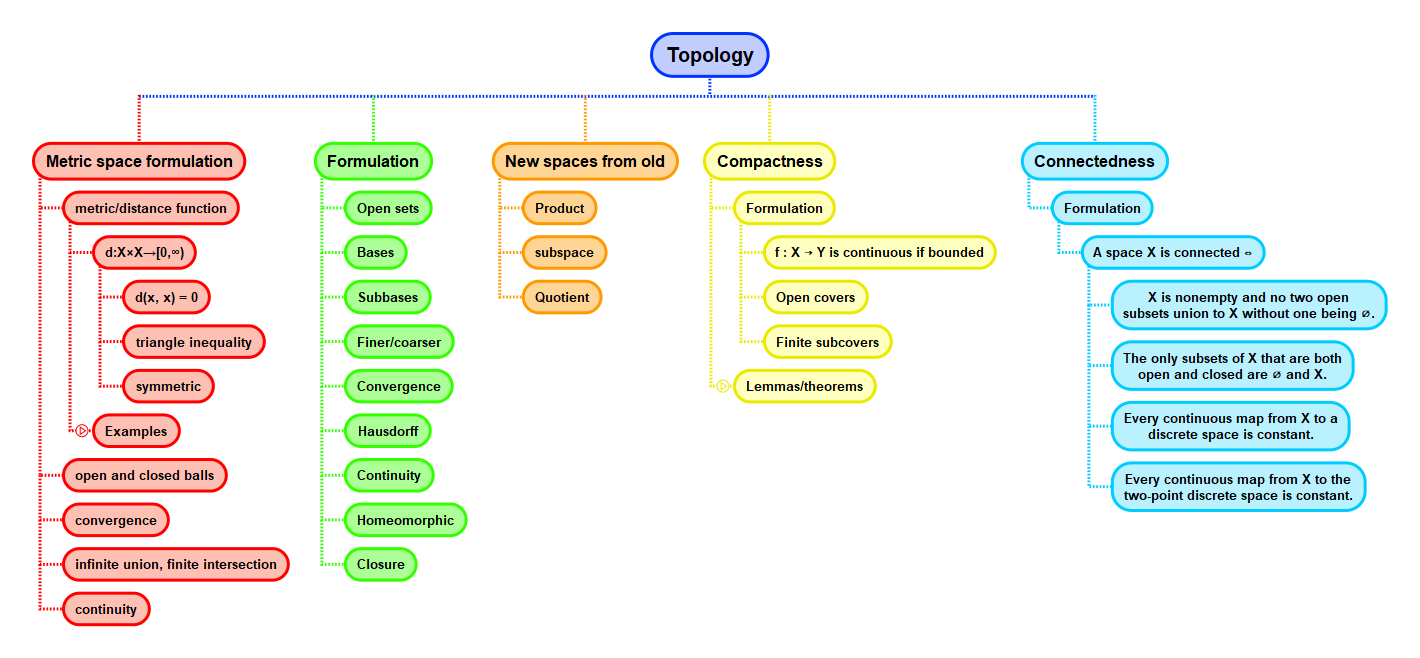Show Question
Math and science::Topology

# Homeomorphisms. Homeomorphic spaces.

### Homeomorphisms and homeomorphic spaces.

Let $$X$$ and $$Y$$ be topological spaces.

Homeomorphism
A homeomorphism from $$X$$ to $$Y$$ is a continuous bijection whose inverse is also continuous.
Homeomorphic spaces
The spaces $$X$$ and $$Y$$ are homeomorphic iff there exists a homeomorphism between them.

$$X \cong Y$$ is the notation for $$X$$ to be homeomorphic to $$Y$$. 'Topologically equivalent' is an alternative term for 'homeomorphic'.

### When are two spaces homeomorphic?

To show that two spaces are homeomorphic, find a homeomorphism between them.

Roughly, two spaces are homeomorphic iff one can be deformed into the other by bending and reshaping, but without tearing or gluing.

### Equivalence relation

Being homeomorphic is an equivalence relation on the class of all topological spaces.

Reflexive
Let $$X$$ be a topological space. Then the identity map on $$X$$ is a homeomorphism.
Symmetric
Let $$f : X \to Y$$ be a homeomorphism. Then $$f^{-1} : Y \to X$$ is a homeomorphism.
Transitive
Let $$f : X \to Y$$ and $$g : Y \to Z$$ be homeomorphisms. Then $$g \circ f : X \to Z$$ is a homeomorphism.

Thus, $$X \cong X$$; $$X \cong Y \iff Y \cong X$$; $$X \cong Y \land Y \cong Z \implies X \cong Z$$.

### Knots homeomorphic to the circle

Some cases of homeomorphisms seem to defy the restriction to bending and reshaping: all knots are homeomorphic to the circle. To see this, take two knots of 1m string; choose a point on each as a starting point; then, trace around each knot at the same rate until you reach the start again. This defines a homeomorphism. While we can't bend a knot into a circle in $$\mathbb{R}^3$$, any knot can be reshaped into a circle if both are first placed in $$\mathbb{R}^4$$.

#### Bijection with non-continuous inverse

The inverse of a continuous bijection need not be continuous.

An easy way of constructing an example is to consider continuous maps to a set with the discrete topology. For example, the identity map $$i : (\mathbb{R}, \text{discrete topology}) \to (\mathbb{R}, \text{standard topology})$$ is continuous, but the inverse,  $$i^{-1} : (\mathbb{R}, \text{standard topology}) \to (\mathbb{R}, \text{discrete topology})$$ is not continuous.

## Context